# 路径规划求最短路径——Dijkstra算法一步一步讲清楚（附代码/可执行）

## 1.最短路径&&Dijkstra算法——引入

（1）定义:从图中的某个顶点出发到达另外一个顶点的所经过的边的权重和最小的一条路径，称为最短路径；

（2）算法应用：移动机器人在路径规划中，得知起始点和终止点，在众多的可行驶路径中，选出最短一条（包含各个节点和各个节点间的距离）；

（3）算法特点：广度优先搜索、赋权有向图（无向图）、单源点（即只存在一个源头点）。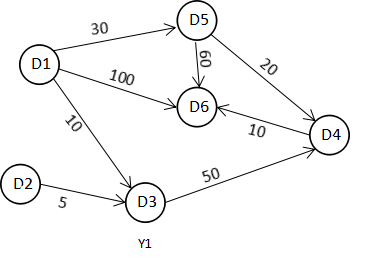E123456
101030100
205
3050
4010
520060
60

①E是一个二维数组，E[i][j]表示边(i,j)的e;

②表格里面的数字是两顶点间的实际长度；

③不相邻的两个点之间的距离是无穷大。

## 2.Dijkstra算法思路

（1）Dijkstra算法是典型最短路径算法，用于计算一个节点到其他所有节点的最短路径。以起始点为中心向外层层扩展，直到扩展到终点为止；

（2）引入两个集合S（dis数组）和U。S的作用是记录已求出最短路径的顶点(以及相应的最短路径长度)，而U则是记录还未求出最短路径的顶点(以及该顶点到起点s的距离)。

## 3.Dijkstra算法操作步骤

（1）初始时，原点 s 的路径权重被赋为 0 （dis[s] = 0）。若对于顶点 s 存在能直接到达的边（s,m），则把dis[m]设为w（s, m）,同时把所有其他（s不能直接到达的）顶点的路径长度设为无穷大。初始时，集合T只有顶点s；

（2）然后，从dis数组选择最小值，则该值就是源点s到该值对应的顶点的最短路径，并且把该点加入到集合S中，OK，此时完成一个顶点；

（3）再然后，我们需要看看新加入的顶点是否可以到达其他顶点并且看看通过该顶点到达其他点的路径长度是否比源点直接到达短，如果是，那么就替换这些顶点在dis中的值；

（4）从dis中找出最小值，重复上述动作，直到S中包含了图的所有顶点。

## 4.Dijkstra算法示例演示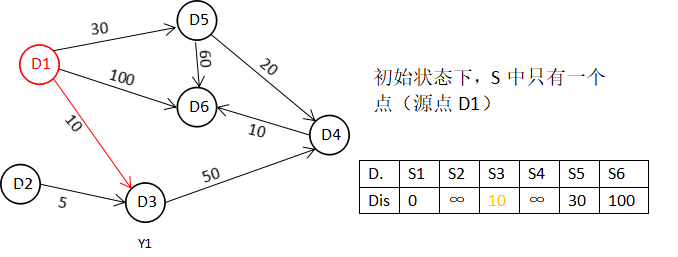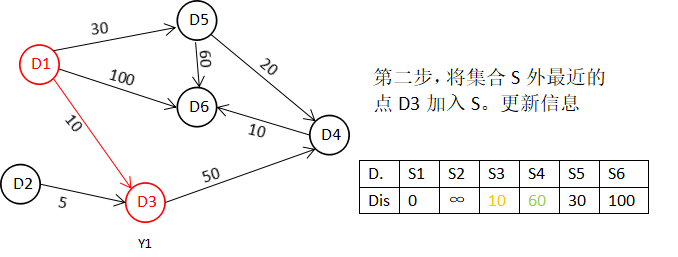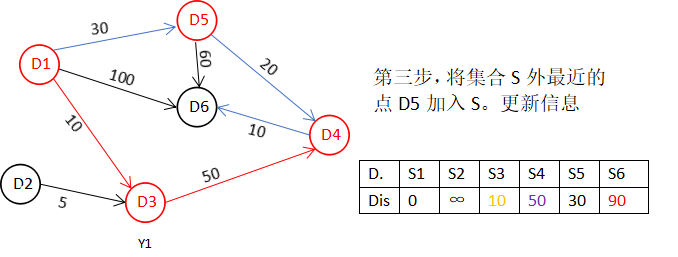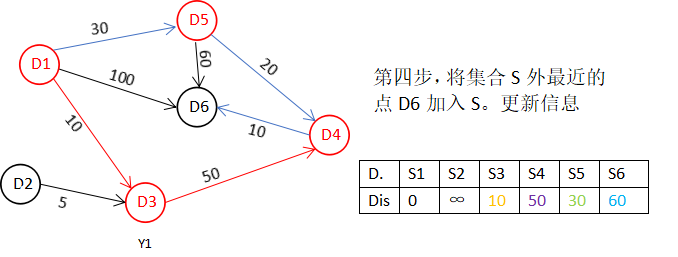D. S1 S2 S3 S4 S5 S6 Dis 0 ∞ 10 50 30 60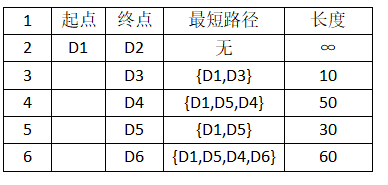## 5.Dijkstra算法的代码解析（1）Dijkstra.h文件的代码

/************************************************************/
/*                程序作者：Willam                          */
/************************************************************/

#pragma once
//保证头文件被编译一次

#include<iostream>
#include<string>
using namespace std;

/*本程序是使用Dijkstra算法来求解最短路径的问题

*/

//记录起点到每个顶点的最短路径的信息
struct Dis
{
string path;
int value;
bool visit;
Dis()
{
visit = false;
value = 0;
path = "";
}
};

class Graph_DG
{
private:
int vexnum;//图的顶点个数
int edge;//图的边数
int **arc;//邻接矩阵
Dis *dis;//记录各个顶点最短路径的信息
public:
//构造函数
Graph_DG(int vexnum, int edge);
//析构函数
~Graph_DG();
//判断我们每次输入的边的信息是否合法
//顶点从1开始编号
bool check_edge_value(int start, int end, int weight);
//创建图
void createGraph();
//打印邻接矩阵
void print();
//求最短路径
void Dijkstra(int begin);
//打印最短路径
void print_path(int);

};

①头文件的作用是用于程序的各种声明，比如这个头文件，就声明了类class Graph_DG{}和结构体struct Dis{}；

②结构体struct Dis{}：记录起点到每个顶点的最短路径的信息；

③类class Graph_DG{}：里面包含了邻接矩阵信息和相关Dijkstra.cpp文件的5个函数的声明；

④采用的邻接矩阵来存储图。

（2）Dijkstra.cpp文件的代码

#include"Dijkstra.h"

//构造函数
Graph_DG::Graph_DG(int vexnum, int edge)
{
//初始化顶点数和边数
this->vexnum = vexnum;
this->edge = edge;
//为邻接矩阵开辟空间和赋初值
arc = new int*[this->vexnum];
dis = new Dis[this->vexnum];
for (int i = 0; i < this->vexnum; i++)
{
arc[i] = new int[this->vexnum];
for (int k = 0; k < this->vexnum; k++)
{
//邻接矩阵初始化为无穷大
arc[i][k] = INT_MAX;
}
}
}

//析构函数
Graph_DG::~Graph_DG()
{
delete[] dis;
for (int i = 0; i < this->vexnum; i++)
{
delete this->arc[i];
}
delete arc;
}

//判断我们每次输入的边的信息是否合法
//顶点从1开始编号
bool Graph_DG::check_edge_value(int start, int end, int weight)
{
if (start<1 || end<1 || start>vexnum || end>vexnum || weight < 0)
{
return false;
}
return true;
}

void Graph_DG::createGraph()
{
cout << "请输入每条边的起点和终点（顶点编号从1开始）以及其权重" << endl;
int start;
int end;
int weight;
int count = 0;
while (count != this->edge)
{
cin >> start >> end >> weight;
//首先判断边的信息是否合法
while (!this->check_edge_value(start, end, weight)) {
cout << "输入的边的信息不合法，请重新输入" << endl;
cin >> start >> end >> weight;
}
//对邻接矩阵对应上的点赋值
arc[start - 1][end - 1] = weight;
//无向图添加上这行代码
//arc[end - 1][start - 1] = weight;
++count;
}
}

void Graph_DG::print() {
cout << "图的邻接矩阵为：" << endl;
int count_row = 0; //打印行的标签
int count_col = 0; //打印列的标签
//开始打印
while (count_row != this->vexnum) {
count_col = 0;
while (count_col != this->vexnum) {
if (arc[count_row][count_col] == INT_MAX)
cout << "∞" << " ";
else
cout << arc[count_row][count_col] << " ";
++count_col;
}
cout << endl;
++count_row;
}
}

void Graph_DG::Dijkstra(int begin) {
//首先初始化我们的dis数组
int i;
for (i = 0; i < this->vexnum; i++) {
//设置当前的路径
dis[i].path = "D" + to_string(begin) + "-->D" + to_string(i + 1);
dis[i].value = arc[begin - 1][i];
}
//设置起点的到起点的路径为0
dis[begin - 1].value = 0;
dis[begin - 1].visit = true;

int count = 1;
//计算剩余的顶点的最短路径（剩余this->vexnum-1个顶点）
while (count != this->vexnum) {
//temp用于保存当前dis数组中最小的那个下标
//min记录的当前的最小值
int temp = 0;
int min = INT_MAX;
for (i = 0; i < this->vexnum; i++) {
if (!dis[i].visit && dis[i].value<min) {
min = dis[i].value;
temp = i;
}
}
//cout << temp + 1 << "  "<<min << endl;
//把temp对应的顶点加入到已经找到的最短路径的集合中
dis[temp].visit = true;
++count;
for (i = 0; i < this->vexnum; i++) {
//注意这里的条件arc[temp][i]!=INT_MAX必须加，不然会出现溢出，从而造成程序异常
if (!dis[i].visit && arc[temp][i] != INT_MAX && (dis[temp].value + arc[temp][i]) < dis[i].value) {
//如果新得到的边可以影响其他为访问的顶点，那就就更新它的最短路径和长度
dis[i].value = dis[temp].value + arc[temp][i];
dis[i].path = dis[temp].path + "-->D" + to_string(i + 1);
}
}
}

}

void Graph_DG::print_path(int begin) {
string str;
str = "D" + to_string(begin);
cout << "以" << str << "为起点的图的最短路径为：" << endl;
for (int i = 0; i != this->vexnum; i++) {
if (dis[i].value != INT_MAX)
cout << dis[i].path << "=" << dis[i].value << endl;
else {
cout << dis[i].path << "是无最短路径的" << endl;
}
}
}

①构造函数Graph_DG::Graph_DG(int vexnum, int edge)：用于设置某些成员变量的初始值；

②析构函数Graph_DG::~Graph_DG()：跳出程序前释放内存；

③bool Graph_DG::check_edge_value(int start, int end, int weight)：判断我们每次输入的边的信息是否合法。顶点从1开始编号，判断起点、终点、权重和图的定点数是否合法；

④void Graph_DG::createGrath()：创建地图；

⑤void Graph_DG::print()：打印地图，打印邻接矩阵；

⑥void Graph_DG::Dijkstra(int begin)：求起点到每个顶点的最短路径；

⑦void Graph_DG::print_path(int begin)：打印各个最短路径。

（3）main.cpp文件的代码

#include"Dijkstra.h"

//检验输入边数和顶点数的值是否有效，可以自己推算为啥：
//顶点数和边数的关系是：((Vexnum*(Vexnum - 1)) / 2) < edge
bool check(int Vexnum, int edge) {
if (Vexnum <= 0 || edge <= 0 || ((Vexnum*(Vexnum - 1)) / 2) < edge)
return false;
return true;
}
int main() {
int vexnum; int edge;

cout << "输入图的顶点个数和边的条数：" << endl;
cin >> vexnum >> edge;
while (!check(vexnum, edge)) {
cout << "输入的数值不合法，请重新输入" << endl;
cin >> vexnum >> edge;
}
Graph_DG graph(vexnum, edge);
graph.createGraph();
graph.print();
graph.Dijkstra(1);
graph.print_path(1);
system("pause");
return 0;
}


①先检验输入边数和顶点数的值是否有效bool check(int Vexnum, int edge)；

②主函数开启：大致流程是先输入输入图的顶点个数和边的条数；

③然后判定它们，如果合法则继续，如果不合法则重新输出；

④调用类Graph_DG graph(vexnum, edge)，引用类里面的四个成员函数，进行处理数据；

⑤暂停/等待用户信号；

⑥返回。

## 6.代码调试结果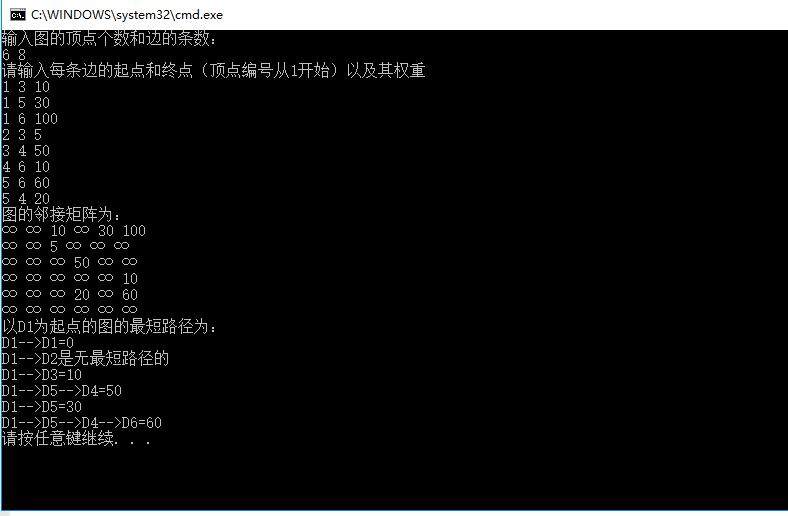12-2403-0857万+
12-19620
03-12106
08-061万+
05-191万+
01-214755
03-3012万+
01-095217
01-084万+
©️2020 CSDN 皮肤主题: 大白 设计师:CSDN官方博客